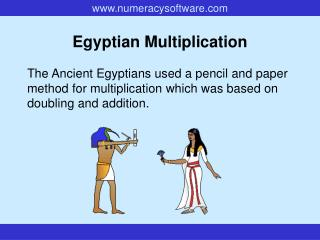# Egyptian Multiplication - PowerPoint PPT PresentationDownload PresentationEgyptian Multiplication

Egyptian MultiplicationDownload Presentation## Egyptian Multiplication

- - - - - - - - - - - - - - - - - - - - - - - - - - - E N D - - - - - - - - - - - - - - - - - - - - - - - - - - -
##### Presentation Transcript

1. Egyptian Multiplication The Ancient Egyptians used a pencil and paper method for multiplication which was based on doubling and addition.

2. 18 x 53 using Egyptian Multiplication 1 53 Write 1 and 53, meaning 1 lot of 53. Work down, doubling the numbers, so that you’ve now got 2, 4, 8, 16, etc. lots of 53. 2 106 4 212 Stop when the number of the left (16) is more than half of the other number you are multiplying (18). 8 424 16 848 Look for numbers on the left that add up to 18 (2 and 16). 954 Cross out the other rows of numbers. Add up the remaining numbers on the right to get the final answer.

3. 76 x 39 using Egyptian Multiplication 1 76 Write 1 and 76, meaning 1 lot of 76. Work down, doubling the numbers, so that you’ve now got 2, 4, 8, 16, etc. lots of 76. 2 152 4 304 Stop when the number of the left (32) is more than half of the other number you are multiplying (39). 8 608 16 1216 Look for numbers on the left that add up to 39 (1, 2, 4 and 32). 32 2432 Cross out the other rows of numbers. 2964 Add up the remaining numbers on the right to get the final answer.

4. Now it’s your turn! This jar holds 17 litres of water.How much water will 26 jars hold? A potter makes 35 pots each week. How many will he make in a year? This chariot travels 23km in an hour. How far will it travel in 45 hours?

5. Now it’s your turn! A soldier marches 28km each day.How far will he march in 38 days? A chest holds 44 large gold coins. How many coins will 35 chests hold? This boat carries 61 soldiers. How many soldiers are there on 26 boats?

6. END OF PRESENTATION